## Assertion and Reason Type Question

Following question consider two statements one labelled as statement-1 and another as statement-2. Study both statements and mark your answer using the codes given blow.
1. Both Statement-1 and Statement-2 are true and Statement-2 is correct explanation of Statement-1.
2. Both Statement-1 and Statement-2 are true and Statement-2 is not a correct explanation of Statement-1.
3. Statement-1 is true but statement-2 is false.
4. Statement-1 is false and statement-2 is true.
Question-1
Statement-1 Free electrons in conductor are always in state of continuous random motion.
Statement-2 The random motion of free electrons is due to thermal energy of the conductor.

Question-2
Statement-1 Drift velocity of electrons in a conductor increase on increasing the temperature of the conductor.
Statement-2 On increasing the temperature of a conductor, resistivity of the conductor increases.

Question-3
Statement-1 For metals relaxation time for electrons decreases with increase in temperature.
Statement-2 With the increase in temperature, number of collision per unit time that electrons made with lattice ion also increases.

(1) a
(2) d
(3) b

## Multiple Choice Type Questions

Question-4
Resistivity of the material of a conductor having uniform area of cross-section varies along its length according to the relation
ρ= ρ0 (a+bx)
if L is the length of the conductor and be the area of cross-section then resistivity of the conductor given by relation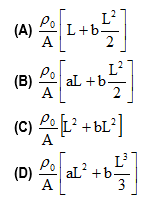Consider an infinitesimally small length element dx of the conductor at a distance x from its one end. Then, the resistance dR of the length element dx of the conductor is
$dR=\rho \frac {dx}{A}= \rho _0 (a+bx) \frac {dx}{A}$
The resistance of the whole length of the conductor is
$R=\int_{0}^{L} dR= \int_{0}^{L} \rho _0 (a+bx) \frac {dx}{A} =\frac {\rho _0 }{A} (aL + \frac {bL^2}{2})$
obtained on solving the integral

Question-5
Given a current carrying wire of non-uniform cross-section. Which of the following is constant through out the wire.
(a)Current only
(b)Current and drift speed
(c)Drift speed only
(d)Current, drift speed and electric field

We know that I = neAvd , Drift Velocity$v_d=\frac {1}{neA} \alpha \frac {1}{A}$ ,
For non-uniform cross-section drift speed will be different for different situations. Only current (rate of flow of charge) will same.

Question-6
When potential difference across a given copper wire is increase, drift velocity of charge carriers
(a)Decreases
(b)Increases
(c)Remain same
(d)Get reduced to zero

We know that
$v_d=\frac {eE}{m} \tau$
If L is the length of the copper wire and V is the potential difference across it then
$v_d=\frac {e}{m} \frac {V}{L} \tau$
$v_d \alpha V$ i.e. it potential difference is increase drift velocity of electrons also increases

Question-7
A material B has twice the specific resistance of the material A. A circular wire made of B has twice the diameter of the wire made of A. Then , for the two wires to have to have the same resistance, the ratio
La/Lb of their respective lengths must be
(a) ½
(b) 2
(c) ¼
(d)1

Let La and Lb be the lengths of two wires.
Given that ρA=ρ i.e. ρB=2ρ also diameter of wire A is DA=D then for wire B DB=2D,
The resistance of the wire made of material A,
$R_A= \frac {\rho _A L_A}{\pi (\frac {D_A}{2})^2} = \frac {\rho _A L_A}{\pi (\frac {D}{2})^2} = \frac {4\rho L_A}{\pi D^2}$
Resistance of the wire made of material B
$R_B= \frac {\rho _B L_B}{\pi (\frac {D_B}{2})^2} = \frac {\rho _B L_B}{\pi (\frac {2D}{2})^2} = \frac {2\rho L_B}{\pi D^2}$
Since RA=RB, we have
$\frac {4\rho L_A}{\pi D^2}=\frac {2\rho L_B}{\pi D^2}$
$\frac {L_A}{L_B} =\frac {1}{2}$

Question-8
What will be the equivalent resistance between A and D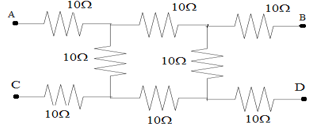(a)40 Ω
(b)20Ω
(c)30Ω
(d)10Ω

Resistance at the end B and C do not be the part of network of resistance between point A and D.
Therefore equivalent resistance between points A and D is
$R= 10 + \frac {1}{10+10} + \frac {1}{10+10} +10$
$R=30 \Omega$

Question-9
In the given circuit below the equivalent resistance between points A and C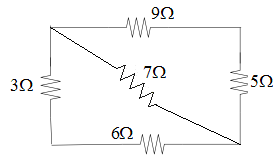(a)32
(b)30.7
(c)33.07
(d)3.07

Resistance of the segment AB and BC are in series. Therefore effective resistance is
$R_1=9+5=14\Omega$
Similarly resistance of the segment AD and DC are in series. IfR2 is the effective resistance
$R_2 =3+6 =9 \Omega$
The resistanceR1 andR2 are in parallel with $7 \Omega$ resistor. Then equivalent resistance is
$\frac {1}{R_eq}= \frac {1}{14} + \frac {1}{9} + \frac {1}{7]$
Req =3.07 $\Omega$

Question-10
A circular ring having negligible resistance is used to connect four resistors as shown below in the figure.
The equivalent resistance between point A and B is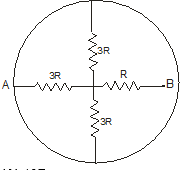(a)10R
(b)2R
(c)3R
(d)R

Above network of resistors is equivalent to the arrangement shown below in the figure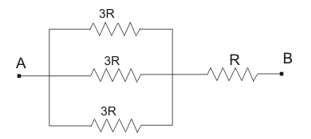If R1 is equivalent resistance of parallel combination then
$\frac {1}{R_1 = \frac {1} {3R} + \frac {1} {3R} + \frac {1} {3R}$
R1 = R
Therefore equivalent resistance of the network between A and B is
Req=R+R=2R

## Paragraph Type Questions

Two concentric metal spherical shells of radius a and b respectively are separated by weakly conducting material of conductivity σ
A potential difference V is applied across the two shells
Question 11
Find the resistance between the shells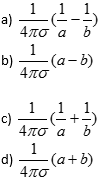Consider a shell of radius r and thickness dr as shown in figure

Resistance of the shell
$dR=\frac{dr}{\sigma4\pi r^2}$

Total resistance

$R=\int_{a}^{b}\frac{dr}{\sigma4\pi r^2}$
$=\frac{1}{4\pi\sigma}(\frac{1}{a}-\frac{1}{b})$

Question 12
Find the electric field at a distance r from the center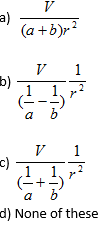We know that
$J=\frac{I}{A}$

And
$J=\sigma E$

So we can say that
$E=\frac{I}{\sigma A}$
Now
$V=IR$
So
$E=\frac{V}{\sigma RA}$
For distance r from center
$E=\frac{V}{\sigma R4\pi r^2}$
Substituting the value of R from above solution
$E=\frac{V}{(\frac{1}{a}-\frac{1}{b})}\frac{1}{r^2}$

## Multiple Choice Type Questions

Question 13
A potential difference of V is applied at the ends of a copper wire of length l and diameter d. On doubling only d, drift velocity
(a) Becomes two times
(b) Becomes half
(c) Does not change
(d) Becomes one fourth

Drift velocity is given by
$v_d=\frac {V}{\rho l ne}$
$v_d$ does not depend upon diameter.
Hence (c) is the correct option

Question 14
The effective resistance between points P and Q of the electrical circuit shown in the figure is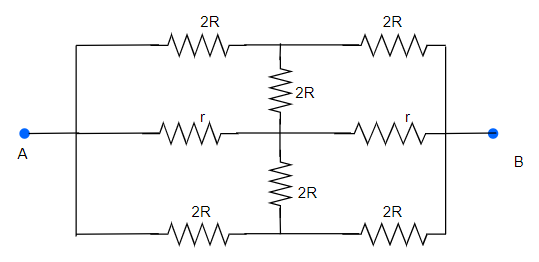(a) $\frac {2Rr}{R+r}$
(b) $8R(R+r)(3R+r)$
(c) $2r+4R$
(d) $\frac {5R}{2} + 2r$

In a circuit, any circuit element placed between points at the same potential can be removed, without affecting the rest of the circuit, here, by symmetry, points P, Q and R are at same potential, for any potential difference between A and B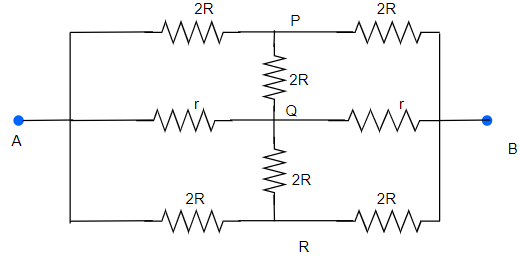The circuit can therefore be reduced as shown below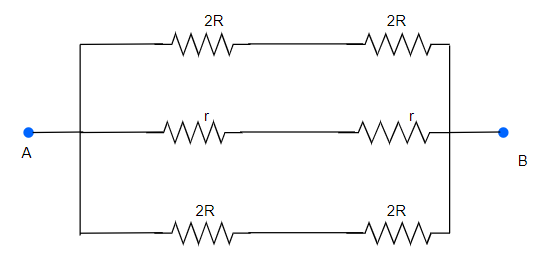Now this can be reduced to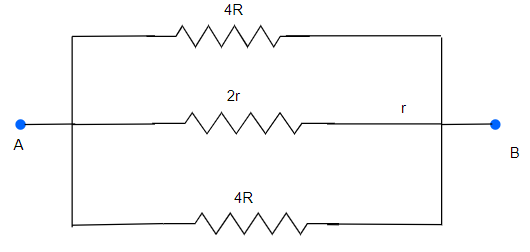Therefore Effective Resistance
$\frac {1}{R_{eq}} = \frac {1}{4R} + \frac {1}{4R} + \frac {1}{2r}$
$\frac {1}{R_{eq}} =\frac {r + r + 2R}{4Rr}$
$R_{eq}=\frac {2Rr}{R+r}$

Question 15
Which of the following characteristics of electrons determines the current in a conductor?
(a) Drift velocity alone.
(b) Thermal velocity alone.
(c) Both drift velocity and thermal velocity.
(d) Neither drift nor thermal velocity.

(a)

Question 16
Water of volume 2 litre in a container is heated with a coil of 1 kW at 27 °C. The lid of the container is open and energy dissipates at rate of 160 J/s. In how much time temperature will rise from 27°C. to 77°C. [Given specific heat of water is 4.2 kJ/kg]
(a) 8 min 20 s
(b) 6 min 2 s
(c) 7 min
(d) 14 min

Heat gained by water = Heat supplied by container - heat lost
$m s \Delta T = 1000t - 160t$
$t= \frac {2 \times 4.2 \times 1000 \time 50}{840}$ = 8 min 20 sec

Question 17
If a wire is stretched to make it 0.1% longer, its resistance will
(a) increase by 0.2%
(b) increase by 0.05%
(c) decrease by 0.2%
(d) decrease by 0.05%

(a)

Question 18
Six identical resistors are connected as shown in the figure. The equivalent resistance will be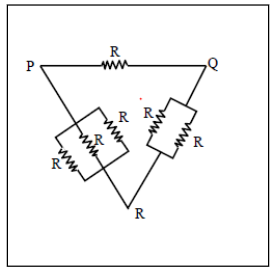(a)Maximum between P and R
(b)Maximum between Q and R
(c)Maximum between P and Q
(d) All are equal

(c)

Question 19
An ideal gas is filled in a closed rigid and thermally insulated container . A coil of 100 ω resistor carrying a current of 1 A for 5 minutes supplies heat to the gas . The change in the internal energy of the gas is
(a) 10 kJ
(b)30 kJ
(c) 20 kJ
(d) 0 kJ

Here,heat supplied = the change in internal energy
$Q= \Delta U$
Now heat supplied $Q=i^2 R t$
$=1 \times 1 \times 100 \times 5 \times 60=30000$ J=30kJ.
Hence (b) is the correct option

Question 20
Temperature dependence of resistivity $\rho(T)$ of semiconductors, insulators and metals is significantly based on the following factors:
(a) number of charge carriers can change with temperature T.
(b) time interval between two successive collisions can depend on T.
(c) length of material can be a function of T.
(d) mass of carriers is a function of T.

(a), (b)